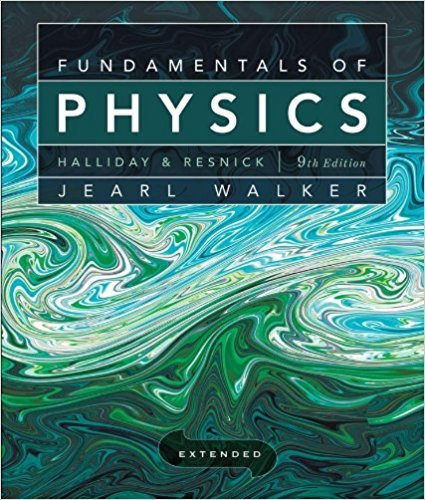×
Log in to StudySoup
Get Full Access to Fundamentals Of Physics Extended - 9 Edition - Chapter 3 - Problem 1
Join StudySoup for FREE
Get Full Access to Fundamentals Of Physics Extended - 9 Edition - Chapter 3 - Problem 1

Already have an account? Login here
×
Reset your password

# Can the sum of the magnitudes of two vectors ever be equalISBN: 9780470469088 189

## Solution for problem 1 Chapter 3

Fundamentals of Physics Extended | 9th Edition

• Textbook Solutions
• 2901 Step-by-step solutions solved by professors and subject experts
• Get 24/7 help from StudySoup virtual teaching assistantsFundamentals of Physics Extended | 9th Edition

4 5 1 332 Reviews
25
2
Problem 1

Can the sum of the magnitudes of two vectors ever be equal to the magnitude of the sum of the same two vectors? If no, why not? If yes, when?

Step-by-Step Solution:
Step 1 of 3

Chapter 28: Physical Optics­ Interference and Diffraction  If two waves occupy the same space, their amplitudes add at each point. They may interfere either constructively or destructively  Conditions for dark fringes (destructive interference) in a two­slit experiment: dsinα = m− 1 λm=1,2,3,… o Above central bright fringe: ( ) 2 1 o Below central bright fringe: inα = ( ) 2 λm=−1,−2,−3,…  Interference is only noticeable if the light sources are monochromatic (so all the light has the same wavelength) and coherent (different sources maintain the same phase relationship over space and time)  Interference will be constructive

Step 2 of 3

Step 3 of 3

##### ISBN: 9780470469088

Unlock Textbook Solution

Enter your email below to unlock your verified solution to:

Can the sum of the magnitudes of two vectors ever be equal